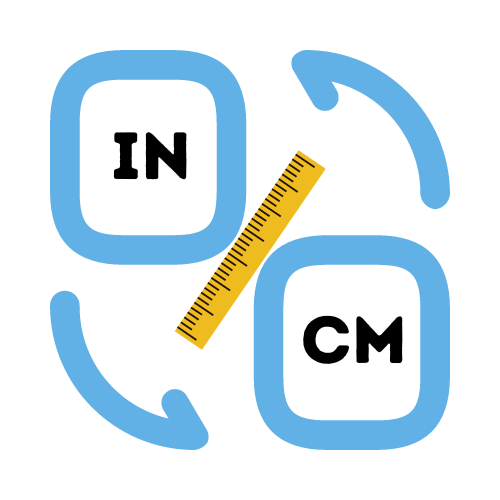# Convert Inches to CM

## Simple conversion of inches to centimeters.

• Created by Emily Rodriguez
• Reviewed by Ramesh Agarwal

Converting between inches and centimeters can be a difficult process, if you don't know what you are doing. Not only do you need to know the equivalent value in both the metric and imperial system, you also need to know which formula to apply to get the desired result.

## How to convert between inches and centimeters

Converting from inches to centimeters is pretty simple and easy to do. Converting between the two units is very easy and you can do it with a simple ruler or tape measure. The inch to cm conversion is just 2.54 cm in 1 inch. To make the conversion more accurate, you can just round it off to the nearest half-inch mark.

For example, if you measure something at 12.75 inches, simply round it off to 13 inches. So if you are looking to convert between inches and centimeters, take a ruler, measure the distance between the two units and divide the number in inches by 2.54.

## How many cm are in an inch?

It is not uncommon to see centimeters and inches used interchangeably. They are both units of measurement for length, but the two vary in terms of size. One inch and one centimetre are not equal in terms of length. To find out how many centimetres there are in one inch, or how many inches are in one centimetre, you need to multiply by the conversion factor.

You are probably wondering how many centimetres in an inch? The important number to remember is 2.54. You will use this number any time you need to convert between cm and inches. There are 2.54 centimeters in an inch, which is one of the most common units of measurement in the world (the other being grams).

The metric system was created in France in the late 1700s and has been used by scientists and mathematicians ever since. Being a decimal system, the metric system is an excellent choice for measuring small or large objects.

## How does an inches to cm converter work?

The fastest way to convert between inches and centimeters is to use a length converter or length calculator tool. Without a doubt, this is both the easiest and fastest way to convert between measurements. This is why we created the helpful length conversion tool.

Simply enter the length in inches and let the calculator instantly convert it to centimeters. Get the results instantly without having to calculate anything else. You can also convert centimeters to inches using the free tool for that too. It works in almost the same way, except you enter the length value in cm and get the appropriate value back in inches.

## Convert inches to cm without a calculator.

If you are wondering how to convert inches to cm without a calculator or special conversion tool, then you are not alone. If you've ever had to convert between inches and centimeters, you know that it can be quite difficult to do without the right equipment. There are many measuring tools that can make the process easy, such as a measuring tape for measuring length. Measuring tapes have markings for both centimeters and inches.

Another method of converting inches to cm is to do it from memory. This works best when you are dealing with measurements that are rounded. For example converting 5 inches into cm. To use this approach, you can remember the most common measurements and their equivalent length in centimeters. See the inches to cm chart below for more info.

However, if you have are only given the measurements in centimeters or inches, then the easiest way to solve this is to use an online calculator or conversion tool. You can use the inches to cm converter on this page. It's quick, easy and available 24/7.

## Inches to CM chart

Knowing the basic length equivalents in both centimeters and inches, can speed up the process of converting between measurements.

We've created a quick reference chart below to simplify the common measurements that we use daily.

Inches to Centimeters Conversion Chart
1 inche in cm = 2.54cm 2 inches in cm = 5.08cm
3 inches in cm = 7.62cm 4 inches in cm = 10.16cm
5 inches in cm = 12.7cm 6 inches in cm = 15.24cm
7 inches in cm = 17.78cm 8 inches in cm = 20.32cm
9 inches in cm = 22.86cm 10 inches in cm = 25.40cm
15 inches in cm = 38.10cm 20 inches in cm = 50.80cm
25 inches in cm = 63.50cm 30 inches in cm = 76.20cm
35 inches in cm = 88.90cm 40 inches in cm = 101.60cm
45 inches in cm = 114.30cm 50 inches in cm = 127.00cm

## What is the inches to cm formula?

An inch has a length that is equal to 2.54cm. However, the length of a centimeter can be converted to 0.393701 inches. Once we know the corresponding length for each unit, we can calculate the length in either inches or cm quite easily.

An example conversion of inches in centimeters:
10 inches in cm, can be calculated as `10 * 2.54 = 25.4 cm`.